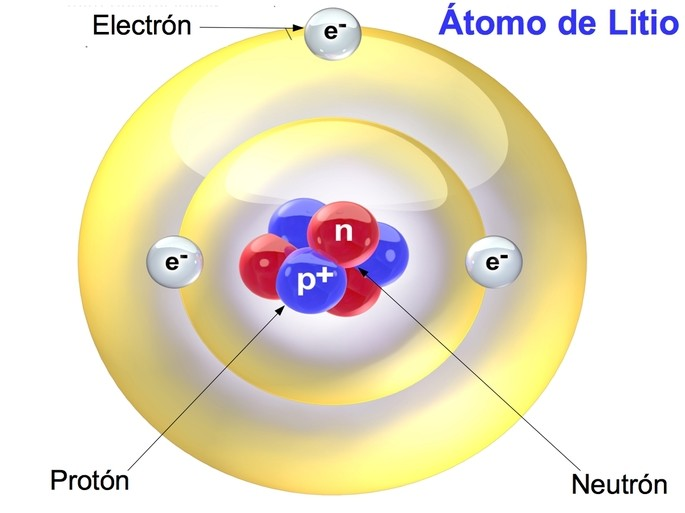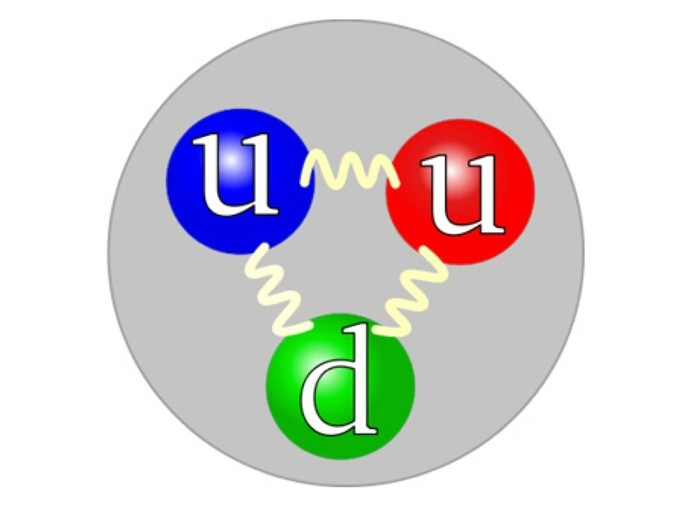# Differences Between Protons, Neutrons, and Electrons

69 / 100
Contents

## Protons, Neutrons, and Electrons: Differences, Definition

Protons, neutrons, and electrons are the particles that make up the atom, which is the smallest part of the element. These particles determine the characteristics and properties of the chemical elements.

The protons and neutrons are concentrated in the atomic nucleus, while the electrons are distributed in the crust or the periphery of the atom. An atom has the same number of electrons and protons, while the number of neutrons is variable.### Protons, Neutrons, and Electrons – What are the Difference?

Next, we present a comparative table between protons, neutrons and electrons.

 Proton Neutron Electron Definition Subatomic particle of positive charge Subatomic Neutral Charge Particle Negatively charged subatomic particle Load Positive +1 Neutral 0 Negative -1 Symbol p + n 0 e – Location in the atom Core Core Peripheral orbitals Mass (kg) 1,673 x 10- -27 kg 1,675 x 10- -27 kg 9,109 x 10- -31 kg Mass compared to proton (amu) 1 1 0.0005 Elemental particle 3 quarks: 2 u and 1 d 3 quarks: 2 d and 1 u 1 lepton Discoverer (year) E. Rutherford (1911) J. Chadwick (1931) JJThomson (1897)

## What is a Proton?

Protons are the positively charged particles found in the nucleus of an atom. Each atom of an element has a fixed number of protons, which determines its atomic number or Z. Thus, hydrogen has one proton and Z equals 1.

The mass of the proton is 1.673 × 10 -27 kg, representing one atomic mass unit or amu (by its acronym atomic mass unit ).

In 1911, Ernest Rutherford discovered that the nucleus of an atom was tiny and positively charged, hence the concept of the proton.

The proton is made up of elementary particles called quarks or quarks: 2 u quarks (by up = up) and 1 quark d (by down = down).Scheme of the quark constitution of a proton, with two quarks “up” (u) and one quarks “down” (d).

### How to calculate the Protons of an atom?

The number of protons in an atom can be determined from its atomic number, which is the position number on the periodic table.

For example, Au gold has an atomic number = 79, which means that gold has 79 protons in its nucleus.

## What is a Neutron?

Neutrons are the particles found in the nucleus of an atom along with protons. They are denoted by the letter n and have a neutral charge. The only element that does not have neutrons is hydrogen.

The mass of neutrons is very similar to that of protons, so the sum of the masses of protons and neutrons determines the atomic mass of an element.

Atoms that have the same atomic number, but different numbers of neutrons are called isotopes. For example, deuterium is an isotope of hydrogen, which has a neutron and a proton in the nucleus.

The English physicist James Chadwick discovered in 1931 this subatomic particle, with a mass close to the proton, but with a neutral electric charge, which is why it was called a neutron.

The neutron is composed like the proton of quarks: 1 quark u (by up = up) and 2 quark d (by down = down).Scheme of the quarks constitution of a neutron, with two quarks “down” (d) and one quarks “up” (u).

### How to calculate the neutrons of an atom?

We can calculate the number of neutrons in an atom if we know its atomic mass and its atomic number Z. We already know that the atomic number Z is the number of protons and the atomic mass is the sum of protons and neutrons that an atom has.

For example, oxygen has an atomic mass of 16 and Z is 8. The number of protons in oxygen equals atomic mass minus Z:

16 – 8 = 8 neutrons

## What is an Electron?

Electrons are the particles of the atom that are in the cloud that surrounds the nucleus. While protons and neutrons are concentrated in the nucleus, the electrons are distributed in layers on the outside.

Electrons in the outermost shell of an atom can jump from one atom to another. This gives the atom a different electrical charge; for example, if an atom gains an electron, its charge will be negative, while if it loses an electron, the charge will be positive.

This is what happens in ions, that is, an atom that gains or loses one or more electrons. For example, chlorine has 17 electrons, but it can gain one electron to become a Cl  chloride anion , with 18 electrons and a negative charge.

The electron was discovered in 1897 by JJ Thomson, the same of the atomic model of “raisin pudding”.

The electron is made up of an elementary particle that is the lepton.

The mass of an electron is almost 2000 times smaller than that of the proton and the neutron. Suppose a proton or neutron were the size of a 10 pound bowling ball, then the electron would be the size of a small marble.

Therefore, the mass of electrons in an atom is negligible when calculating the atomic mass.

### How to calculate the Electrons of an atom?

The number of electrons in a neutral atom is equal to the number of protons in that atom. For example, Au gold has 79 protons, therefore it will have 79 electrons.